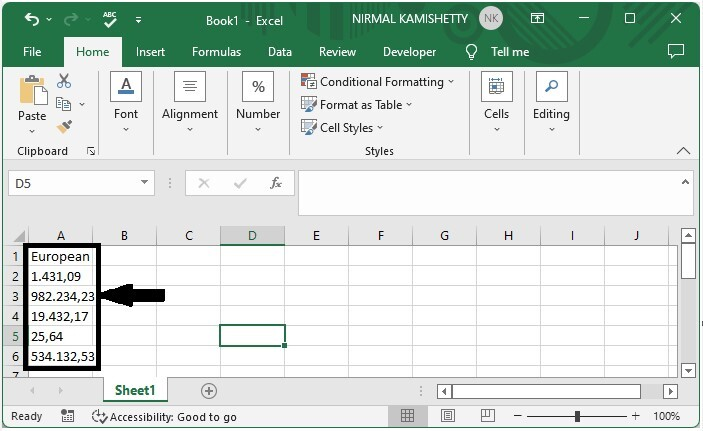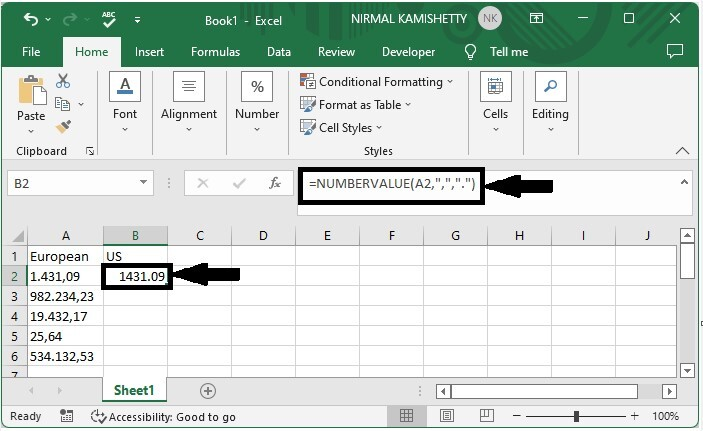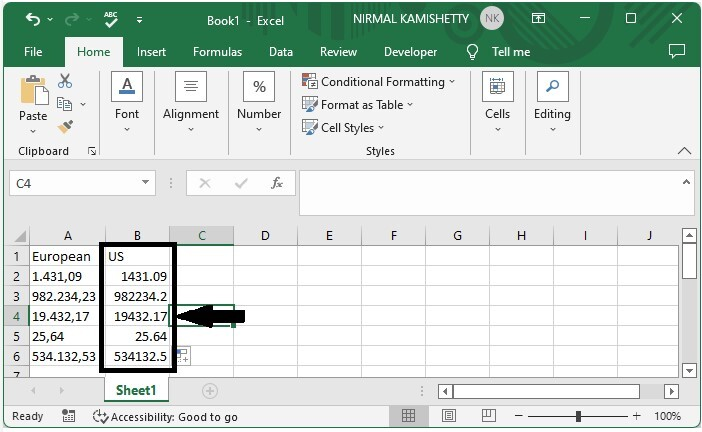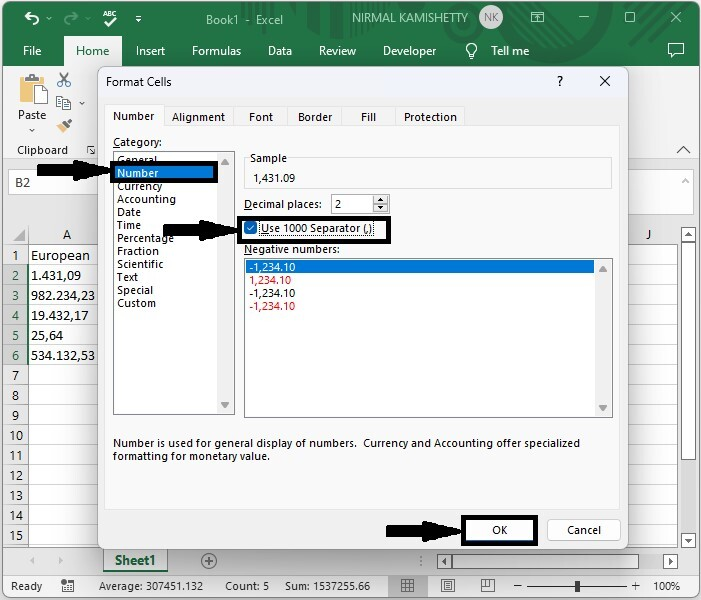# How to Convert Number Format between European and US in Excel?

Generally, in Excel, when using the US format, the numbers will be like 3432.12, but in the European format, the same number will be typed as 3432.12. This can occasionally lead to misunderstanding. So, we need to convert US format to European format for more accurate analysis and simplicity. Read this tutorial to learn how you can convert number formats in Excel between European and American.

## Converting Number Format from European to US in Excel

Here we will use the NUMBERVALUE formula to get any one of the results, then use the autofill handle to get all the results. Let's take a look at a simple procedure for converting number formats in Excel between European and American.

### Step 1

Let us consider an Excel sheet that contains a list of numbers in European format, similar to the below image.To get our first result, click on an empty cell, in this case cell B2, and enter the formula =NUMBERVALUE(A2, "," ,"." )  and press enter. In the formula, A2 is the address of the values in the sheet.

Empty cell > Formula > Enter### Step 2

Then drag down from the first result using the auto-fill handle to fill all the values as shown in the below image.### Step 3

Then, select the new values and right-click, then click on format cells, then click on number in the new pop-up, then check the box labelled "use the separator," and finally click OK to finish our process.

Select values > Right click > Format cells > Number > Check box > OKNote − We can also use the formula as =SUBSTITUTE(SUBSTITUTE(A2, ".", "" ), ",", ".")+0.

If we need to convert a US number to a European format, we can use the formula as follows −

=SUBSTITUTE(SUBSTITUTE(SUBSTITUTE(FIXED(A2,2,FALSE),".","$"),",","."),"$",",") and there will be no need to format cells.

## Conclusion

In this tutorial, we used a simple example to demonstrate how you can convert number formats between European and American in Excel.

Updated on: 06-Mar-2023

2K+ Views